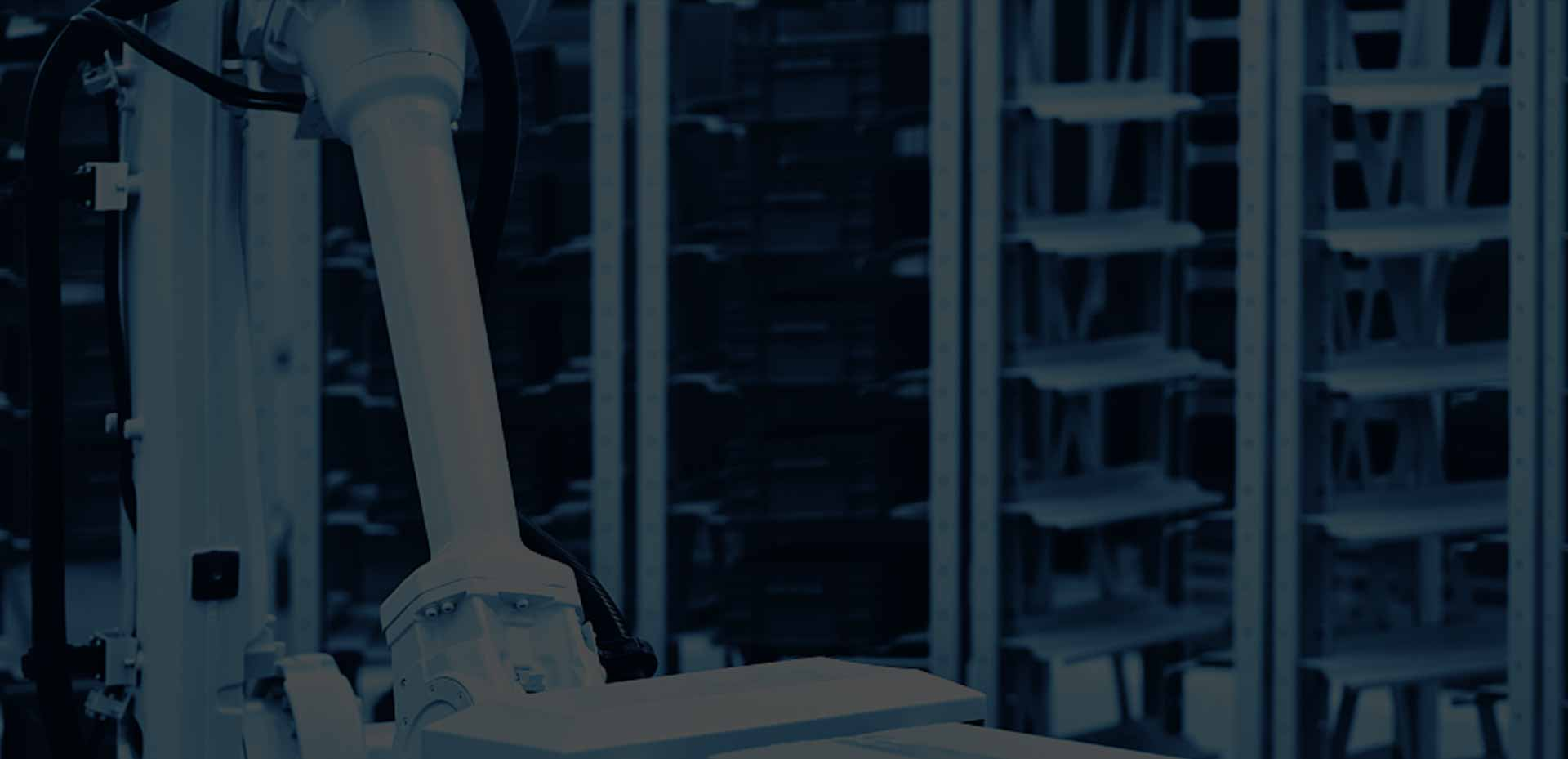﻿ Five Performance Parameters of Pressure Sensors Are Explained - Anhui Tianguang Sensor Co., Ltd.Make Weighing Easier
TG Load Cells: Make Weighing Easier

Call Us

86-552-4923688

Englishx
Make Weighing Easier
• En
MAKE FORCE SENSORS SAFER AND SMARTER
Widely used in various occasions requiring force measurement and process monitoring.

# Five Performance Parameters of Pressure Sensors Are Explained

Pressure sensor is a device that can feel the pressure and convert the pressure change into an electrical signal output, is the most common kind of sensor in the automation equipment, but also the automatic force measurement equipment in the nervous system. The correct use of pressure sensors must first understand the pressure sensor parameters.

Pressure sensors mainly have the following parameters:

1. Range

Range refers to the rated load of the pressure sensor for sale. The general unit is KGf, N, etc. Such as the range of 100KGf, the sensor measurement range that is 0-100KGf.

2. Sensitivity

Sensitivity is the output signal coefficient of the pressure sensor, the unit is mV / V, common 1mV / V, 2mV / V, the full range of the pressure sensor output = working voltage * sensitivity, example: working voltage 5VDC, sensitivity 2mV / V, the full range of output that is 5V * 2mV / V = 10mV, such as the full range of the pressure sensor for 100KG, pressure full 100KG, the output That is 10mV, the pressure of 50KG that is 5mV.

3. Nonlinearity

Non-linearity is the percentage of the maximum deviation between the output value of the empty load and the output value of the rated load determined by the straight line and the measured curve of the increased load for the rated output value. Theoretically, the output of the sensor should be linear, but in fact it is not, non-linearity is the percentage of deviation from the ideal. Non-linear units: %FS, non-linear error = range * non-linear, such as the range of 100KG, non-linear for 0.05% FS, non-linear error that is: 100KG * 0.05% = 0.05KG.

4. Repeatability

5. Creep

Creep refers to the load remains unchanged, the other test conditions also remain unchanged, the pressure sensor output change over time on the percentage of rated output, generally taken 30min.

NEXT: NO_INFORMATIONGet in Touch
•